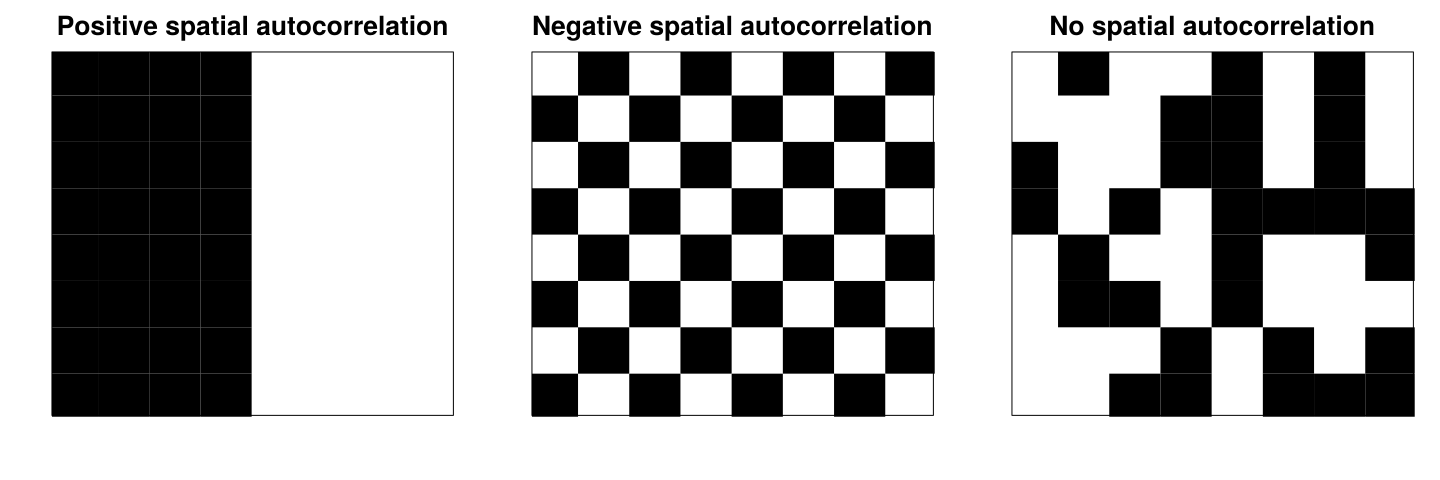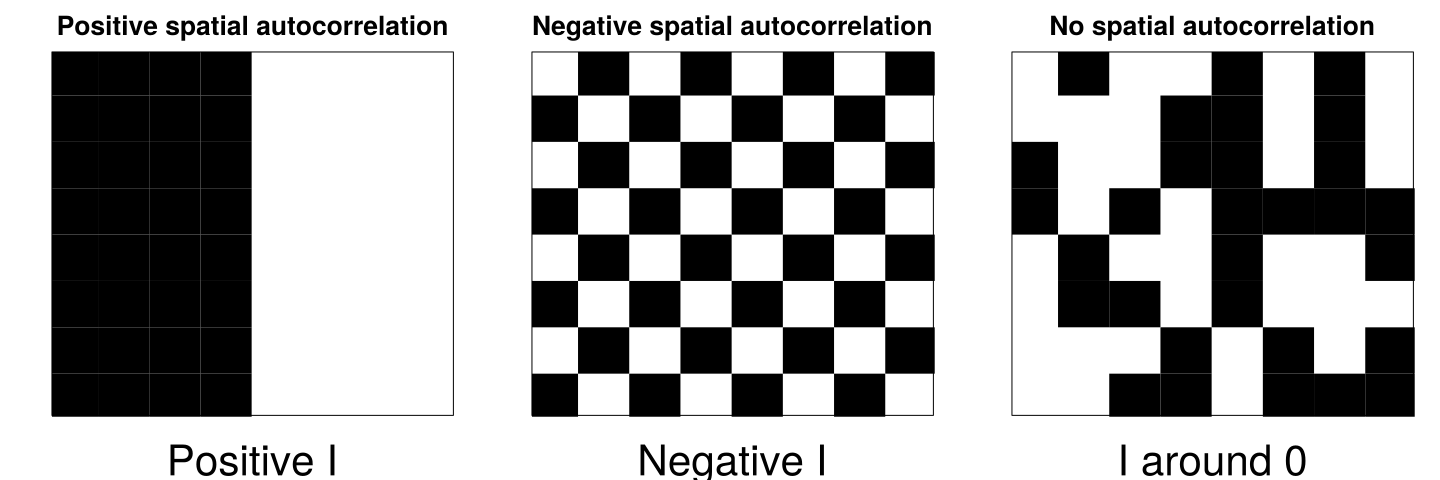# A brief introduction to spatial autocorrelation

## What is spatial autocorrelation?

• Spatial structures play important roles in the analysis of ecological data. Living communities are spatially structured at many spatial scales (Borcard, Gillet, and Legendre (2011)).

• The environmental elements such as climatic, physical, chemical forces control living communities. If these factors are spatially structured, their patterns will reflect on the living communities.
• Example: patches in the desert where the soil is humid enough to support vegetation.

## Spatial autocorrelation

According to the geographer Waldo R. Tobler, the first law of geography is:

Everything is related to everything else, but near things are more related than distant things.

Spatial autocorrelation can causes problems for statistical methods that make assumptions about the independence of residuals.

## Spatial autocorrelation

Spatial data can be positively spatially autocorrelated, negatively spatially autocorrelated, or not (or randomly) spatially autocorrelated.

A positive spatial autocorrelation means that similar values are close to each other.

A negative spatial autocorrelation means that similar values are distant from each other.

A random spatial autocorrelation means that, in general, similar values are neither close nor distant from each other.## Moran’s index

The Moran’s index (Moran’s I) is widely used to measure spatial autocorrelation based on feature locations and feature values simultaneously.

$\begin{equation} I = \frac{n}{S_0} \frac{\displaystyle\sum_{i=1}^n \sum_{j=1}^n w_{ij}(x_i - \bar{x})(x_j - \bar{x})}{\displaystyle\sum_{i=1}^n (x_i - \bar{x})^2} \label{eq:morani} \end{equation}$ where $$w_{ij}$$ is the weight between observation $$i$$ and $$j$$, and $$S_0$$ is the sum of all $$w_{ij}$$’s.

## Moran’s index

Moran’s I can vary between -1 and 1 (like a normal correlation index).## Moran’s I

There are two types of Moran’s I:

1. Global Moran’s I is a measure of the overall spatial autocorrelation.
2. Local Moran’s I is a measure of the local spatial autocorrelation (e.x.: locally at each station).

## A working example

We will use bird diversity data (https://bit.ly/2BLOwdd) to learn how to deal with spatial autocorrelation.

df <- read_table2("data/bird.diversity.txt")  # Load tidyverse first
df <- janitor::clean_names(df)  # Clean column names

df
## # A tibble: 64 x 5
##     site bird_diversity tree_diversity lon_x lat_y
##    <dbl>          <dbl>          <dbl> <dbl> <dbl>
##  1     1           7.18           4.12 -118.  33.7
##  2     2           7.54           6.12 -117.  34.1
##  3     3           4.89           4.1  -118.  34.0
##  4     4           4.15           4.8  -118.  33.8
##  5     5           5.90           3.8  -118.  33.9
##  6     6           4.72           3.7  -118.  33.7
##  7     7           3.16           4.07 -119.  33.7
##  8     8           4.05           5    -118.  33.7
##  9     9           7.27           4.2  -118.  34.1
## 10    10           6.53           4.9  -118.  33.9
## # … with 54 more rows

## Tree diversity explains bird diversity

There is indeed a positive influence of tree diversity on bird diversity.

ggplot(df, aes(x = tree_diversity, y = bird_diversity)) +
geom_point(size = 3) + geom_smooth(method = "lm")